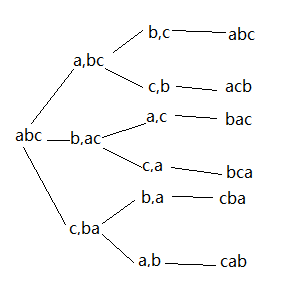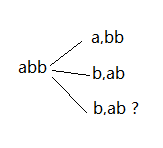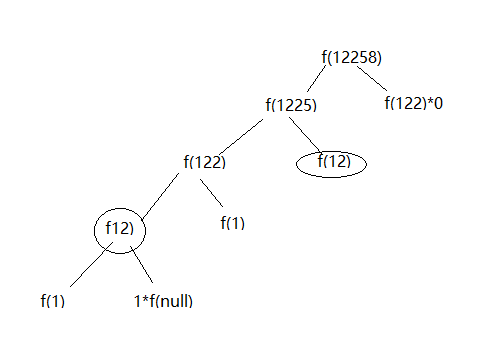### 深度优先搜索f(12258) = g(8)f(1225) + g(58)f(122)，g()判断能不能被翻译，g(8)=1, g(58)=0f(0)=1;
f(1)=1;
f(2)=f(1)+0f(0)=1,g(58)=0;
f(3)=f(2)+1
f(1)=2,g(25)=1;
f(4)=f(3)+1f(2)=3,g(22)=1;
f(5)=f(4)+1
f(3)=5,g(12)=1;